Home Practice
For learners and parents For teachers and schools
Textbooks
Full catalogue
Pricing SupportLog in

We think you are located in United States. Is this correct?

# 2.2 Momentum

## 2.2 Momentum (ESCJ7)

Momentum is a physical quantity which is closely related to forces. Momentum is a property which applies to moving objects, in fact it is mass in motion. If something has mass and it is moving then it has momentum.

Momentum

The linear momentum of a particle (object) is a vector quantity equal to the product of the mass of the particle (object) and its velocity.

The momentum (symbol $$\vec{p}$$) of an object of mass $$m$$ moving at velocity $$v$$ is:

$\vec{p}=m\vec{v}$

Momentum is directly proportional to both the mass and velocity of an object. A small car travelling at the same velocity as a big truck will have a smaller momentum than the truck. The smaller the mass, the smaller the momentum for a fixed velocity. If the mass is constant then the greater the velocity the greater the momentum. The momentum will always be in the same direction as the velocity because mass is a scalar not a vector.

temp text

### Vector nature of momentum (ESCJ8)

A car travelling at $$\text{120}$$ $$\text{km·hr^{-1}}$$ will have a larger momentum than the same car travelling at $$\text{60}$$ $$\text{km·hr^{-1}}$$. Momentum is also related to velocity; the smaller the velocity, the smaller the momentum.

Different objects can also have the same momentum, for example a car travelling slowly can have the same momentum as a motorcycle travelling relatively fast. We can easily demonstrate this.

Consider a car of mass $$\text{1 000}$$ $$\text{kg}$$ with a velocity of $$\text{8}$$ $$\text{m·s^{-1}}$$ (about $$\text{30}$$ $$\text{km·hr^{-1}}$$) East. The momentum of the car is therefore:

\begin{align*} \vec{p}& = m\vec{v} \\ & = \left(\text{1 000}\right)\left(8\right) \\ & = \text{8 000}\text{ kg·m·s$^{-1}$}~\text{East} \end{align*}

Now consider a motorcycle, also travelling East, of mass $$\text{250}$$ $$\text{kg}$$ travelling at $$\text{32}$$ $$\text{m·s^{-1}}$$ (about $$\text{115}$$ $$\text{km·hr^{-1}}$$). The momentum of the motorcycle is:

\begin{align*} \vec{p} &= m\vec{v} \\ & = \left(250 \text{kg}\right)\left(32 \text{m·s$^{-1}$}\right) \\ & = \text{8 000}\text{ kg·m·s$^{-1}$}~\text{East} \end{align*}

Even though the motorcycle is considerably lighter than the car, the fact that the motorcycle is travelling much faster than the car means that the momentum of both vehicles is the same.

From the calculations above, you are able to derive the unit for momentum as $$\text{kg·m·s^{-1}}$$.

Momentum is also vector quantity, because it is the product of a scalar ($$m$$) with a vector $$(\vec{v})$$.

A vector multiplied by a scalar has the same direction as the original vector but a magnitude that is scaled by the multiplicative factor.

This means that whenever we calculate the momentum of an object, we should include the direction of the momentum.

## Worked example 1: Momentum of a soccer ball

A soccer ball of mass $$\text{420}$$ $$\text{g}$$ is kicked at $$\text{20}$$ $$\text{m·s^{-1}}$$ towards the goal post. Calculate the momentum of the ball.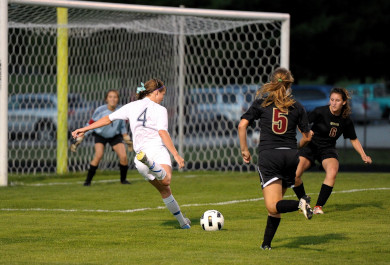### Identify what information is given and what is asked for

The question explicitly gives:

• the mass of the ball, and

• the velocity of the ball.

The mass of the ball must be converted to SI units.

$$\text{420}\text{ g}=\text{0,42}\text{ kg}$$

We are asked to calculate the momentum of the ball. From the definition of momentum, $$\vec{p}=m\vec{v}$$ we see that we need the mass and velocity of the ball, which we are given.

### Do the calculation

We calculate the magnitude of the momentum of the ball,

\begin{align*} \vec{p}& = m\vec{v} \\ & = \left(\text{0,42}\right)\left(20\right) \\ & = \text{8,40}\text{ kg·m·s$^{-1}$} \end{align*}

We quote the answer with the direction of motion included, $$\vec{p}$$ = $$\text{8,40}$$ $$\text{kg·m·s^{-1}}$$ in the direction of the goal post.

## Worked example 2: Momentum of a cricket ball

A cricket ball of mass $$\text{160}$$ $$\text{g}$$ is bowled at $$\text{40}$$ $$\text{m·s^{-1}}$$ towards a batsman. Calculate the momentum of the cricket ball.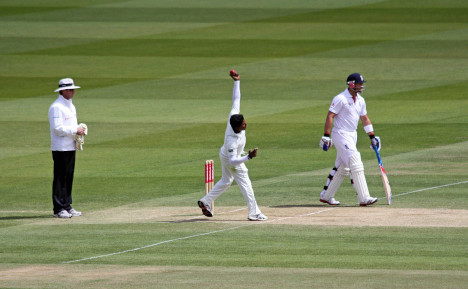### Identify what information is given and what is asked for

The question explicitly gives

• the mass of the ball (m = $$\text{160}$$ $$\text{g}$$ = $$\text{0,16}$$ $$\text{kg}$$), and

• the velocity of the ball $$(\vec{v}$$ = $$\text{40}$$ $$\text{m·s^{-1}}$$ towards the batsman)

To calculate the momentum we will use

$$\vec{p}=m\vec{v}.$$

### Do the calculation

\begin{align*} \vec{p}& = m\vec{v} \\ & = \left(\text{0,16}\right)\left(40\right) \\ & = \text{6,4}\text{ kg·m·s$^{-1}$} \\ & = \text{6,4}\text{ kg·m·s$^{-1}$} \text{in the direction of the batsman} \end{align*}

The momentum of the cricket ball is $$\text{6,4}$$ $$\text{kg·m·s^{-1}}$$ in the direction of the batsman.

## Worked example 3: Momentum of the Moon

The centre of the Moon is approximately $$\text{384 400}$$ $$\text{km}$$ away from the centre of the Earth and orbits the Earth in $$\text{27,3}$$ days. If the Moon has a mass of $$\text{7,35} \times \text{10}^{\text{22}}$$ $$\text{kg}$$, what is the magnitude of its momentum (using the definition given in this chapter) if we assume a circular orbit? The actual momentum of the Moon is more complex but we do not cover that in this chapter.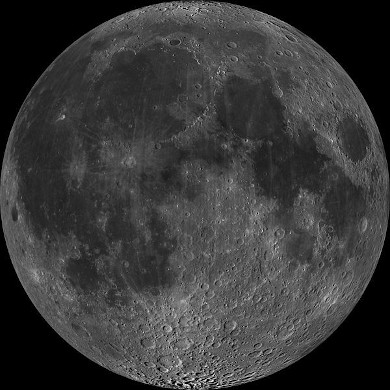### Identify what information is given and what is asked for

The question explicitly gives

• the mass of the Moon ($$m$$ = $$\text{7,35} \times \text{10}^{\text{22}}$$ $$\text{kg}$$)

• the distance to the Moon ($$\text{384 400}$$ $$\text{km}$$ = $$\text{384 400 000}$$ $$\text{m}$$ = $$\text{3,844} \times \text{10}^{\text{8}}$$ $$\text{m}$$)

• the time for one orbit of the Moon ($$\text{27,3} \text{ days} = \text{27,3} \times 24 \times 60 \times 60 = \text{2,36} \times \text{10}^{\text{6}}\text{ s}$$)

We are asked to calculate only the magnitude of the momentum of the Moon (i.e. we do not need to specify a direction). In order to do this we require the mass and the magnitude of the velocity of the Moon, since

$$\vec{p}=m\vec{v}.$$

### Find the magnitude of the velocity of the Moon

The magnitude of the average velocity is the same as the speed. Therefore:

$$v=\frac{\Delta x}{\Delta t}$$

We are given the time the Moon takes for one orbit but not how far it travels in that time. However, we can work this out from the distance to the Moon and the fact that the Moon has a circular orbit. Using the equation for the circumference, C, of a circle in terms of its radius, we can determine the distance travelled by the Moon in one orbit:

\begin{align*} C& = 2\pi r \\ & = 2\pi \left(\text{3,844} \times \text{10}^{\text{8}}\right) \\ & = \text{2,42} \times \text{10}^{\text{9}}\text{ m} \end{align*}

Combining the distance travelled by the Moon in an orbit and the time taken by the Moon to complete one orbit, we can determine the magnitude of the Moon's velocity or speed,

\begin{align*} v& = \frac{\Delta x}{\Delta t} \\ & = \frac{C}{T} \\ & = \frac{\text{2,42} \times \text{10}^{\text{9}}\text{ m}}{\text{2,36} \times \text{10}^{\text{6}}\text{ s}} \\ & = \text{1,02} \times \text{10}^{\text{3}}\text{ m·s$^{-1}$} \end{align*}

### Finally calculate the momentum and quote the answer

The magnitude of the Moon's momentum is:

\begin{align*} \vec{p}& = m\vec{v} \\ {p}& = m{v} \\ & = \left(\text{7,35} \times \text{10}^{\text{22}}\right)\left(\text{1,02} \times \text{10}^{\text{3}}\right) \\ & = \text{7,50} \times \text{10}^{\text{25}}\text{ kg·m·s$^{-1}$} \end{align*}

The magnitude of the momentum of the Moon is $$\text{7,50} \times \text{10}^{\text{25}}$$ $$\text{kg·m·s^{-1}}$$.

As we have said, momentum is a vector quantity. Since momentum is a vector, the techniques of vector addition discussed in Vectors and scalars in Grade 10 must be used when dealing with momentum.

temp text

### Change in momentum (ESCJ9)

Particles or objects can collide with other particles or objects, we know that this will often change their velocity (and maybe their mass) so their momentum is likely to change as well. We will deal with collisions in detail a little bit later but we are going to start by looking at the details of the change in momentum for a single particle or object.

#### Case 1: Object bouncing off a wall

Lets start with a simple picture, a ball of mass, $$m$$, moving with initial velocity, $$\vec{v}_i$$, to the right towards a wall. It will have momentum $$\vec{p}_i=m\vec{v}_i$$ to the right as shown in this picture:The ball bounces off the wall. It will now be moving to the left, with the same mass, but a different velocity, $$\vec{v}_f$$ and therefore, a different momentum, $$\vec{p}_f=m\vec{v}_f$$, as shown in this picture:We know that the final momentum vector must be the sum of the initial momentum vector and the change in momentum vector, $$\Delta \vec{p}=m\Delta \vec{v}$$. This means that, using tail-to-head vector addition, $$\Delta \vec{p}$$, must be the vector that starts at the head of $$\vec{p}_i$$ and ends on the head of $$\vec{p}_f$$ as shown in this picture: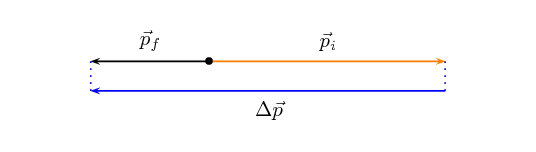We also know from algebraic addition of vectors that: \begin{align*} \vec{p}_f &=\vec{p}_i + \Delta \vec{p} \\ \vec{p}_f - \vec{p}_i &= \Delta \vec{p} \\ \Delta \vec{p} &= \vec{p}_f - \vec{p}_i \end{align*} If we put this all together we can show the sequence and the change in momentum in one diagram: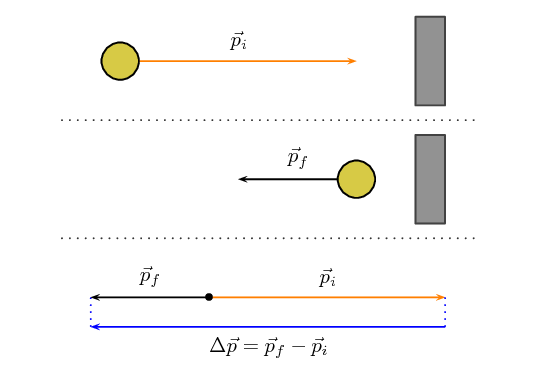We have just shown the case for a rebounding object. There are a few other cases we can use to illustrate the basic features but they are all built up in the same way.

#### Case 2: Object stops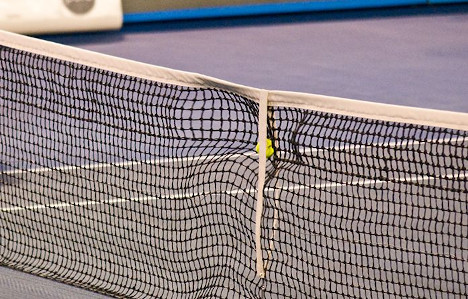In some scenarios the object may come to a standstill (rest). An example of such a case is a tennis ball hitting the net. The net stops the ball but doesn't cause it to bounce back. At the instant before it falls to the ground its velocity is zero. This scenario is described in this image: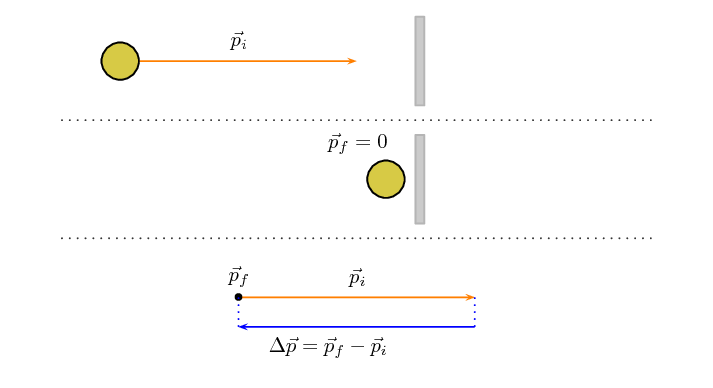#### Case 3: Object continues more slowly

In this case, the object continue in the same direction but more slowly. To give this some context, this could happen when a ball hits a glass window and goes through it or an object sliding on a frictionless surface encounters a small rough patch before carrying on along the frictionless surface.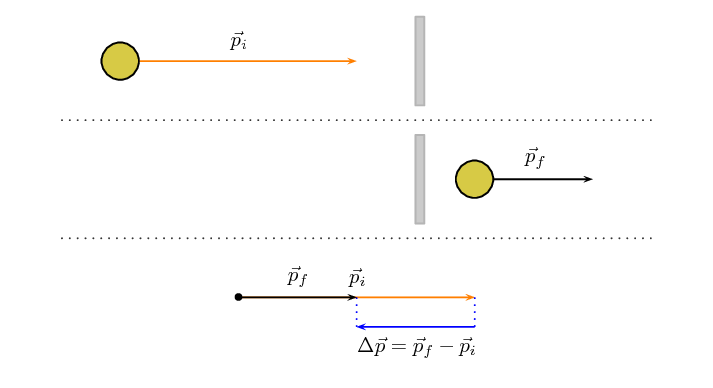Important: note that even though the momentum remains in the same direction the change in momentum is in the opposite direction because the magnitude of the final momentum is less than the magnitude of the initial momentum.

#### Case 4: Object gets a boost

In this case the object interacts with something that increases the velocity it has without changing its direction. For example, in squash the ball can bounce off a back wall towards the front wall and a player can hit it with a racquet in the same direction, increasing its velocity.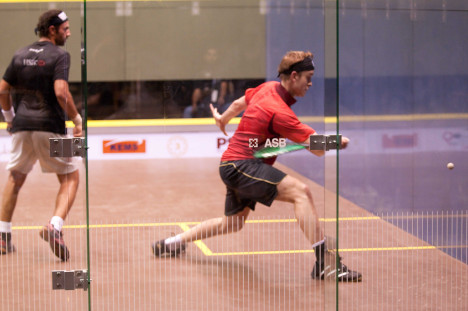If we analyse this scenario in the same way as the first 3 cases, it will look like this: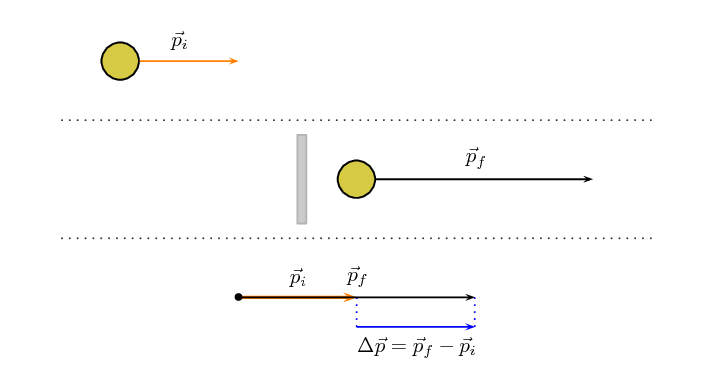#### Case 5: Vertical bounce

For this explanation we are ignoring any effect of gravity. This isn't accurate but we will learn more about the role of gravity in this scenarion in the next chapter.

All of the examples that we've shown so far have been in the horizontal direction. That is just a coincidence, this approach applies for vertical or horizontal cases. In fact, it applies to any scenario where the initial and final vectors fall on the same line, any 1-dimensional (1D) problem. We will only deal with 1D scenarios in this chapter. For example, a stationary basketball player bouncing a ball.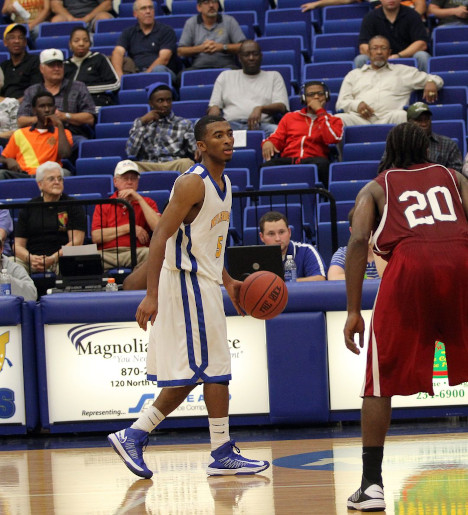To illustrate the point, here is what the analysis would look like for a ball bouncing off the floor: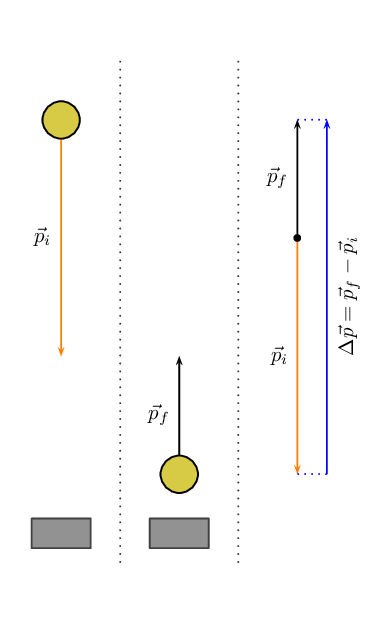Textbook Exercise 2.1

The fastest recorded delivery for a cricket ball is $$\text{161,3}$$ $$\text{km·hr^{-1}}$$, bowled by Shoaib Akhtar of Pakistan during a match against England in the 2003 Cricket World Cup, held in South Africa. Calculate the ball's momentum if it has a mass of $$\text{160}$$ $$\text{g}$$.

$p = mv$

$$v = \text{161,3}\text{ km·hr^{-1}}$$ and $$m = \text{160}\text{ g}$$.

Converting the velocity to the correct S.I units:

\begin{align*} v & = \text{161,3}\text{ km·hr$^{-1}$} \times \frac{\text{1 000}\text{ m}}{\text{3 600}\text{ s}} \\ & = \text{44,81}\text{ m·s$^{-1}$} \end{align*}

Converting the mass to the correct S.I units:

\begin{align*} m & = \text{160}\text{ g} \times \frac{\text{1}\text{ kg}}{\text{1 000}\text{ g}} \\ & = \text{0,16}\text{ kg} \end{align*}

Therefore, computing the momentum:

\begin{align*} p & = mv \\ & = (\text{0,16})(\text{44,81}) \\ & = \text{7,17}\text{ kg·m·s$^{-1}$} \end{align*}

The fastest tennis service by a man is $$\text{246,2}$$ $$\text{km·hr^{-1}}$$ by Andy Roddick of the United States of America during a match in London in 2004. Calculate the ball's momentum if it has a mass of $$\text{58}$$ $$\text{g}$$.

$p = mv$

$$v = \text{246,2}\text{ km·hr^{-1}}$$ and $$m = \text{58}\text{ g}$$.

Converting the velocity to the correct S.I units:

\begin{align*} v & = \text{246,2}\text{ km·hr$^{-1}$} \times \frac{\text{1 000}\text{ m}}{\text{3 600}\text{ s}} \\ & = \text{68,39}\text{ m·s$^{-1}$} \end{align*}

Converting the mass to the correct S.I units:

\begin{align*} m & = \text{58}\text{ g} \times \frac{\text{1}\text{ kg}}{\text{1 000}\text{ g}} \\ & = \text{0,058}\text{ kg} \end{align*}

Therefore, computing the momentum:

\begin{align*} p & = mv \\ & = (\text{0,058})(\text{68,39}) \\ & = \text{3,97}\text{ kg·m·s$^{-1}$} \end{align*}

The fastest server in the women's game is Venus Williams of the United States of America, who recorded a serve of $$\text{205}$$ $$\text{km·hr^{-1}}$$ during a match in Switzerland in 1998. Calculate the ball's momentum if it has a mass of $$\text{58}$$ $$\text{g}$$.

$p = mv$

$$v = \text{205}\text{ km·hr^{-1}}$$ and $$m = \text{58}\text{ g}$$.

Converting the velocity to the correct S.I units:

\begin{align*} v & = \text{205}\text{ km·hr$^{-1}$} \times \frac{\text{1 000}\text{ m}}{\text{3 600}\text{ s}} \\ & = \text{56,94}\text{ m·s$^{-1}$} \end{align*}

Converting the mass to the correct S.I units:

\begin{align*} m & = \text{58}\text{ g} \times \frac{\text{1}\text{ kg}}{\text{1 000}\text{ g}} \\ & = \text{0,058}\text{ kg} \end{align*}

Therefore, computing the momentum:

\begin{align*} p & = mv \\ & = (\text{0,058})(\text{56,94}) \\ & = \text{3,30}\text{ kg·m·s$^{-1}$} \end{align*}

If you had a choice of facing Shoaib, Andy or Venus and didn't want to get hurt, who would you choose based on the momentum of each ball?

The ball with the smallest momentum gives you the least chance of being hurt and so you would choose to face Venus.